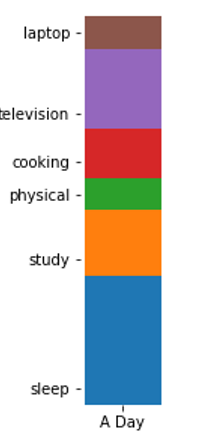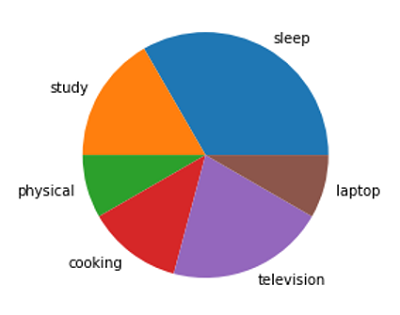# Python | Bar Distribution Plot vs Pie Plot

In this tutorial, we are going to compare bar distribution plot and pie plot using matplotlib in Python?
Submitted by Anuj Singh, on August 12, 2020## Python code for bar distribution plot vs pie plot

```#Data Visualization using Python

import numpy as np
import matplotlib.pyplot as plt

N = 8

xx = np.array(['A Day'])
y = [8,4,2,3,5,2]
yy = np.array([0,8,12,14,17,22])
labl = ['sleep', 'study', 'physical', 'cooking', 'television', 'laptop']

# Bar Distributed Plot
plt.figure(figsize=(1,5))
plt.bar(xx, y, bottom=yy, width=0.02, label=labl)
plt.bar(xx, y, bottom=yy, width=0.02, label=labl)
plt.bar(xx, y, bottom=yy, width=0.02, label=labl)
plt.bar(xx, y, bottom=yy, width=0.02, label=labl)
plt.bar(xx, y, bottom=yy, width=0.02, label=labl)
plt.bar(xx, y, bottom=yy, width=0.02, label=labl)
plt.box(False)
plt.yticks(ticks=yy+1, labels=labl)
plt.xticks(None)
plt.show()

# Pie Plot
plt.figure()
plt.pie(y, labels=labl)
plt.axis(False)
plt.show()
```

Output:

```Output is as Figure
```

Languages: » C » C++ » C++ STL » Java » Data Structure » C#.Net » Android » Kotlin » SQL
Web Technologies: » PHP » Python » JavaScript » CSS » Ajax » Node.js » Web programming/HTML
Solved programs: » C » C++ » DS » Java » C#
Aptitude que. & ans.: » C » C++ » Java » DBMS
Interview que. & ans.: » C » Embedded C » Java » SEO » HR
CS Subjects: » CS Basics » O.S. » Networks » DBMS » Embedded Systems » Cloud Computing
» Machine learning » CS Organizations » Linux » DOS
More: » Articles » Puzzles » News/Updates